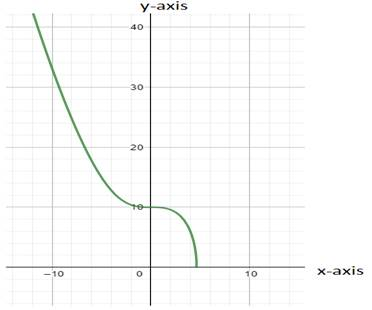# Viewing rectangle from which we can draw appropriate graph of the function.### Precalculus: Mathematics for Calcu...

6th Edition
Stewart + 5 others
Publisher: Cengage Learning
ISBN: 9780840068071### Precalculus: Mathematics for Calcu...

6th Edition
Stewart + 5 others
Publisher: Cengage Learning
ISBN: 9780840068071

#### Solutions

Chapter 2, Problem 46RE
To determine

## To find: Viewing rectangle from which we can draw appropriate graph of the function.

Expert Solution

### Explanation of Solution

Given information:

f(x)=100x3

Calculation:

If f(x) to be defined

100x30x3100x(100)13x464

Hence we can write domain of function as (,4.64] and range of function is [0,)So in given options required viewing rectangle to draw most appropriate graph is [4,4]by[4,4] because viewing rectangle should be lie inside domain of function.

Interpretation:

Viewing rectangle for most appropriate graph is [4,4]by[4,4]

### Have a homework question?

Subscribe to bartleby learn! Ask subject matter experts 30 homework questions each month. Plus, you’ll have access to millions of step-by-step textbook answers!